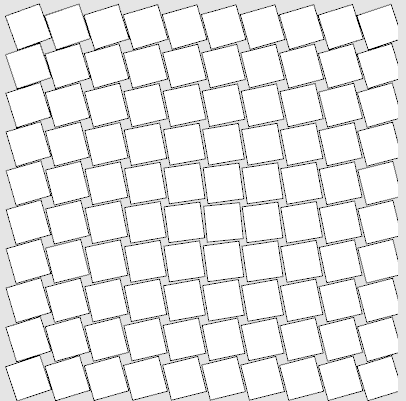## sketch experiment 5 - grid

For my next sketch experiment I placed some rectangles on a grid and rotated them but delayed the rotation by the distance of the center.this is the code I used to create the sketch - I probably should have transofrmed the distance calculation into a function now that I think of it. well - maybe next time :-)

``````(defsketch blub (
(title "sketch ex5")
(width 410)
(height 410)
(f 0)
(p 0)
)
(incf f 0.1)
(background (rgb .9 .9 .9))

(dotimes (i 10)
(dotimes (j 10 )
(setf dx (- 5 i))
(setf dy (- 5 j))
(setf p (- f (* 2 (sqrt (+ (* dx dx) (* dy dy))))))
(push-matrix)
(translate (+ 30 (* i 40)) (+ 25 (* j 40)))
(rotate p)
(rect -19 -19 36 36)
(pop-matrix)
)
)
)
``````